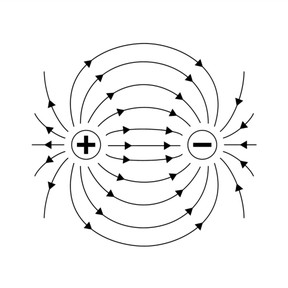Introduction to electricity

# Introduction to electricity

I can describe how charged electric particles can exert forces on one another.

No account needed.8,000 schools use Gynzy92,000 teachers use Gynzy1,600,000 students use Gynzy

## General

Students are introduced to electricity and learn about charged particles and the science of electric force.

NGSS: MS-PS2-3

## Learning objective

Students will be able to describe how charged particles can exert forces on one another.

## Introduction

Start the lesson with an Electricity investigation. Hand out balloons to the students in class and have them blow up the balloons. (optional- you can have blown up balloons and hand those out in class to save time). Have students follow the instructions given and see what happens to their hair as they gently rub the balloon against their hair and then hold the balloon away. Have the students discuss hypotheses about what happened with their partners. Explain that the faint pulling they felt (and saw) on their hair was caused by static electricity.

## Instruction

Tell students that static electricity is one kind of electricity and that it is all an electric force. On the next slide, review what an atom is and what its parts are called. You need to know what an atom is and its parts are to be able to understand electricity. In particular, for electricity we need to focus on the two charged particles, protons and electrons. Explain that protons have a positive charge, and electrons have a negative charge. These charged particles can exert electric forces on one another- depending on how far apart they are the force is stronger or weaker. Show students that two objects with the same charge have a repulsive electric force, pushing them apart, whereas two objects with opposite charges have an attractive force, pulling them together. Next show students the diagram of the electric field and explain that these lines show the force of the (invisible) electric field around a charged object. Have students form pairs to answer the next questions and review the learning thus far. Continue with discussing how larger objects get charged, and explain that this is done by the movement and transfer of electrons. Teach students the three main ways of charging an object: friction, conduction, and induction. For each type of charge, define how the charge is created and give an example as shown on the interactive whiteboard.

## Quiz

Students are given true or false questions to check understanding of key concepts taught within the lesson. They are then asked to determine if the electric charge will increase or decrease when given a diagram to consider. Finally they are asked to determine which charges exhibit an attractive force and what is exchanged to create electric charge.

## Closing

Discuss that students have now learned how charged electric particles can exert forces on one another and how this is the first step in understanding the electricity all around us. Close by asking students to complete an exit slip answering the two questions on the interactive whiteboard.

## Teaching tips

For students who get confused between electrons and protons, you can teach them the alliterative trick that proton & positive both start with P which means that electron and negative are leftover together.

## Instruction materials

- balloons (1 per group/pair or one for each student)

### The online teaching platform for interactive whiteboards and displays in schools

• Save time building lessons

• Manage the classroom more efficiently

• Increase student engagement